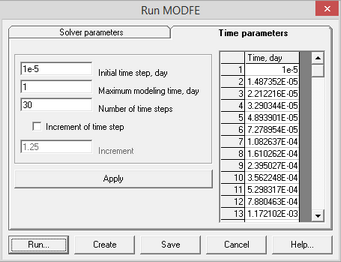﻿ Numerical modeling (ANSRADIAL) > Running MODFE program > Time parameters

# Time parameters

The "Time parameters" tab of "Run MODFE" dialog box allows to specify time discretization parameters for calculation.

The dialog box is launched from the main menu "Numerical models > MODFE > Run MODFE"."Run MODFE" dialog: time parameters.

Explanatory table

 "Initial time step" text box Initial time step for modeling "Maximal modeling time" text box Total modeling time "Number of time steps" text box The number of time steps "Increment for time step" option Allows to use the increment value for time step calculation "Increment" text box Time step increment value "Apply" button Calculates time steps and displays them in a table. When "Increment for time step" option is switched on, steps are calculated using the increment value; when it is switched off the logarithmic discretization from the initial step through the maximal time value is applied Table Shows the time step number and time (in days), for which the calculation is made

Button functions see in the "Solver parameters" tab.